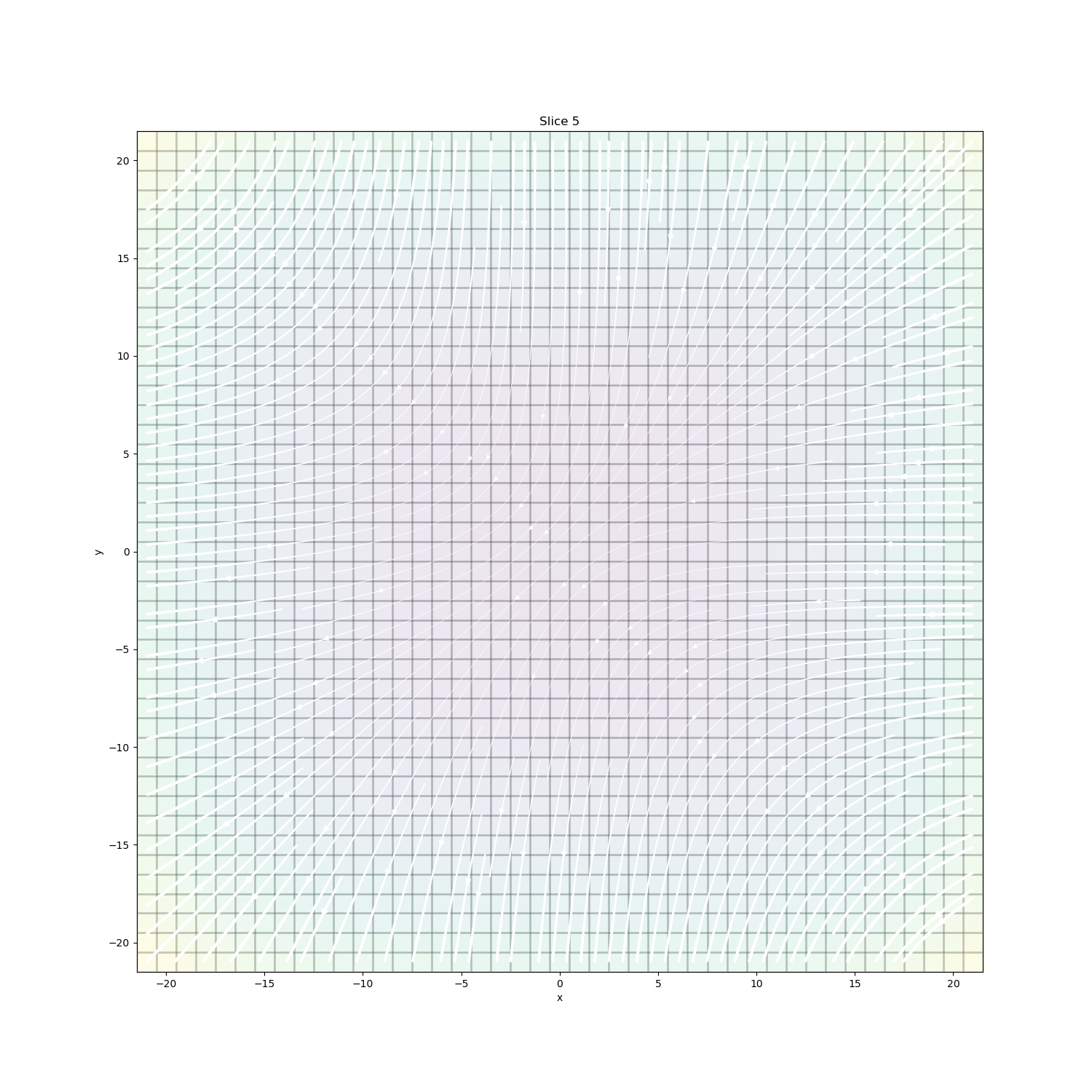# Plotting: Streamline thickness#

A simple example to vary streamline thickness based on the vector amplitudes

Author: @micmitch <https://github.com/micmitch>

```import numpy as np
import matplotlib.pyplot as plt

from discretize import TensorMesh
```

## Create mesh#

Minimum cell size in each direction

```dx = 1.0
dy = 1.0
dz = 1.0

# Number of core cells in each direction
nCoreX = 43.0
nCoreY = 43.0
nCoreZ = 43.0

# Cell widths
hx = [(dx, nCoreX)]
hy = [(dy, nCoreY)]
hz = [(dz, nCoreZ)]

# Desired Core mesh origin (Bottom SW corner)
x0 = -21.5
y0 = -21.5
z0 = -21.5

mesh = TensorMesh([hx, hy, hz], [x0, y0, z0])
print(mesh.nC)
```
```79507
```

## Define arbitrary function to plot#

```X = mesh.gridCC[:, 0]
Y = mesh.gridCC[:, 1]
Z = mesh.gridCC[:, 2]

U = -1 - X ** 2 + Y + Z
V = 1 + X - Y ** 2 + Z
W = 1 + X + Y - Z ** 2
```

## Plot streamlines#

Create figure

```fig = plt.figure()
ax = plt.subplot(111)
fig.set_figheight(15)
fig.set_figwidth(15)
labelsize = 30.0
ticksize = 30.0

# Create data vector
dataVec = np.hstack([U, V, W])
print(dataVec.shape)

# Set streamline plotting options
stream_opts = {"color": "w", "density": 2.0}
pcolor_opts = {"cmap": "viridis"}

dat = mesh.plotSlice(
dataVec,
ax=ax,
normal="Z",
ind=5,
v_type="CCv",
view="vec",
stream_opts=stream_opts,
grid_opts={"color": "k", "alpha": 0.1},
grid=True,
clim=None,
stream_thickness=3,
)
``````(238521,)
```

## Moving Forward#

If you have suggestions for improving this example, please create a pull request on the example in discretize

Total running time of the script: ( 0 minutes 0.989 seconds)

Gallery generated by Sphinx-Gallery